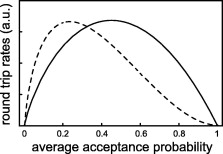## Optimal temperature ladders in replica exchange simulations

Chem. Phys. Letters, 2009, 473, 193-195 published on 29.04.2009
Chem. Phys. Lett.

In replica exchange simulations, a temperature ladder with N rungs spans a given temperature interval. Considering systems with heat capacities independent of the temperature, here we address the question of how large N should be chosen for an optimally fast diffusion of the replicas through the temperature space. Using a simple example we show that choosing average acceptance probabilities of about 45% and computing N accordingly maximizes the round trip rates r across the given temperature range. This result differs from previous analyses which suggested smaller average acceptance probabilities of about 23%. We show that the latter choice maximizes the ratio r/N instead of r.